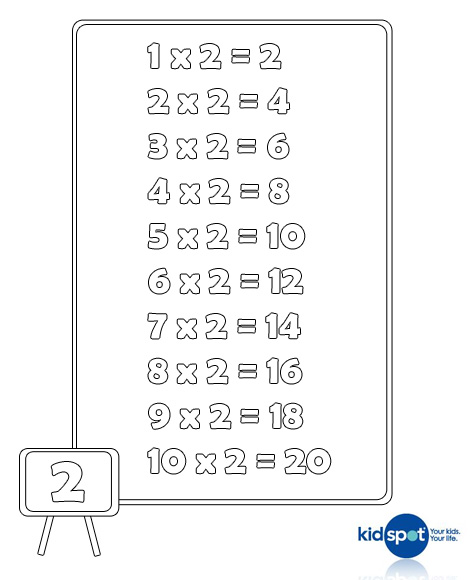# Times tables for kids: 2 times tables

There are many ways to learn the two times tables. Counting the houses on the "even" side of the street is one way, but a printable times table that kids can colour and cut and paste into charts is another. Get them learning the fun way by printing these two times tables.

What you need:

• A printer
• This printable chart of the two times tables
• Pencils to colour in

Number of players:

Activity:

Memorising multiplication times tables is now considered old-fashioned as an educational tool. Yet there's no doubt that "knowing your times tables" can be helpful, even if it is just to give a child the confidence to deliver a rote answer when they are older!

There are many methods to teach children multiplication concepts, but the most important thing is that your child has the ability to visualise the multiplication process before times tables can truly be understood. Children can start to understand mathematical concepts by using toys or food to see how adding and taking away truly "looks". This can be further reinforced by letting children have fun with numbers and maths concepts.

Colouring in, doing dot-to-dots and mazes are all helpful learning games which allow children to explore the fun of numbers, times tables and mathematics.Click here to print the two times tables multiplication chart out.

### Tips for teaching kids multiplication

Multiplication is taught from the first year of school which can surprise parents as many think of multiplication as a concept taught later in primary school. In fact the strong foundations for multiplication are taught from kindergarten or prep.

If your child is aged five to six years then they will be focusing on modelling equal groups or rows of items. They are using the language ‘group’ to describe a collection of items. This ability to ‘see’ the groups is important.

Children aged seven to eight years can count by ones, twos, fives and tens in rhythmic patterns. They learn that equal rows are called arrays. Your child will also be making the connection that three groups of two gives the same answer as two groups of three but that it looks different. These are the basis of multiplication concepts.

Children aged 11 and 12 are multiplying three and four digit numbers by one digit as well as long multiplication which is multiplying three digits by two digits. Accuracy with times tables is assumed at this age as students should have a level of automaticity which allows them to move onto problem solving skills.